# Time-Series Learning using Monotonic Logical Properties

• Marcell Vazquez-Chanlatte$$^{\star (\text{me})}$$
• Shromona Ghosh$$^\star$$
• Jyotirmoy V. Deshmukh$$^{\dagger}$$
• Alberto Sangiovanni-Vincentelli$$^{\star}$$
• Sanjit A. Seshia$$^\star$$

Slides @ mjvc.me/RV2018

$$\star$$: University of California-Berkeley

$$\dagger$$: University of Southern California

# The Verification Problem# Specification Mining# An Incomplete Survery

Survey: Li, Wenchao. Specification mining: New formalisms, algorithms and applications.$^1$Vazquez-Chanlatte et al, Logic-based Clustering and Learning for Time-Series Data, CAV 2017

$^2$ Bombara et al., Signal Clustering Using Temporal Logics, RV 2017.

# Two Messages

## Logical Lens

This talk is about unifying these two.

# Contributions

1. Relax restrictions of our CAV 2017 work.
2. Learn "traffic slow-down" spec from simple logical lens.

## Logical Lens$$^*$$ $\downarrow$ Logic Respecting Distance or Features

$$^*$$Logical Lens = A Parametrized family of Boolean Properties, $$\phi : \text{Parameter} \to \big (\text{Data} \to \{0, 1\}\big )$$, e.g. PSTL.

# In memory of Oded Maler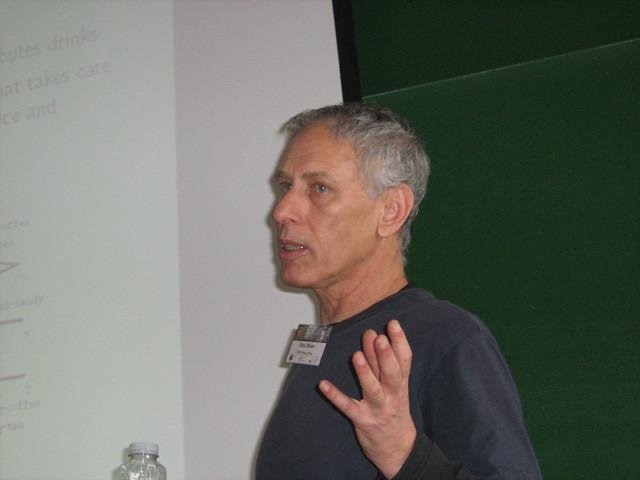• Overlapped with my internship at Toyota.
• Work on "Learning Monotone Partitions of Partially-Ordered Domains" was catalyst for this paper.

# Outline

1. Motivating Example
2. Logical Lens $$\to$$ Logical Distance

Logical Lens $$\triangleq$$ Parametric Trace Property

3. Computing Logical Distance
4. Application to Highway 101 data.

# Motivating Example

Goal: Detect highway slow downs.Have rough idea of shapes to look for.

# Outline

1. Motivating Example
2. Logical Lens $$\to$$ Logical Distance

Logical Lens $$\triangleq$$ Parametric Trace Property

3. Computing Logical Distance
4. Application to Highway 101 data.

# Parametric Logical Formula

$\phi(\tau, h) \triangleq \square_{[\tau, 20]}(\text{car_speed} \leq h)$Different parameters result in different classifications.

# Platonic Ideal of a Metric

If two things are logically similar, they should be "close" together.

# Platonic Ideal of a Metric

If two things are logically similar, they should be "close" together.

"If it quacks like a duck and looks like a duck$$\ldots$$ it should have a small distance to a duck."

# Domain Independent

Time-Series using PSTL is just an example# Logically Similar# Boolean Features

$\varphi_i : \text{Data} \to \{0, 1\}$Each axis represents a property.

# Families of Boolean features

• Sometimes need to test $\geq 3$ properties.
• Consider the following (infinite) size family of Boolean features.You must be $h$ meters tall or more to ride.

# Families of Boolean features

$\phi : \text{Height} \to \bigg ( \text{Data} \to \{0, 1\}\bigg)$# Families of Boolean features

$\phi : \text{Data} \to \bigg ( \text{Height} \to \{0, 1\}\bigg)$# Parametric Specifications

$\phi : \text{Data} \to \bigg ( {[0, 1]}^n \to \{0, 1\}\bigg)$

Data induces a geometric structure in the parameter space.# Monotonic Specifications

$\phi : \text{Data} \to \bigg ( {[0, 1]}^n \to \{0, 1\}\bigg)$

1. Complicated geometry in general.
2. Many families are "monotonic".$\theta \leq \theta' \implies \phi(x)(\theta) \leq \phi(x)(\theta')$

# Can Forget Interior

It is sufficent to just remember the boundary (surface)# Logical Lens

Map Data to Surfaces.CAV 2017 work can only pick out a single point.

# 💰Billion Dollar Question💰

Does similar data induce a similar structure??1. Transform data into a surface encoding logical trade-offs.
2. Does this transformation give us a metric that achieves our goals?

# Platonic Ideal 2.0

"If it quacks like a duck and looks like a duck$$\ldots$$ it should produce a similar validity domain boundary??"

Does similar data induce a similar structure??

It depends...

For many Monotonic Specifications this is true: $\big( x \geq \theta\big ) \text{ vs } \big(x + 0.1 \geq \theta\big)$# Logical Distance Recipe

1. Define logical lens: $\phi : \text{Data} \to \bigg ( {[0, 1]}^n \to \{0, 1\}\bigg)$
2. Define distance metric between parameters.
3. Lift parameter distance to distance between boundaries. (e.g., Hausdorff Distance)

# Outline

1. Motivating Example
2. Logical Lens $$\to$$ Logical Distance

Logical Lens $$\triangleq$$ Parametric Trace Property

3. Computing Logical Distance
4. Application to Highway 101 data.

# Recursively Computing Boundaries

1.2. Sample points from each rectangle.
3. Compute Hausdorff Distance.

Technique due to Oded Maler.

Oded Maler. Learning Monotone Partitions of Partially-Ordered Domains 2017. 〈hal-01556243〉

# Recursively Computing Boundaries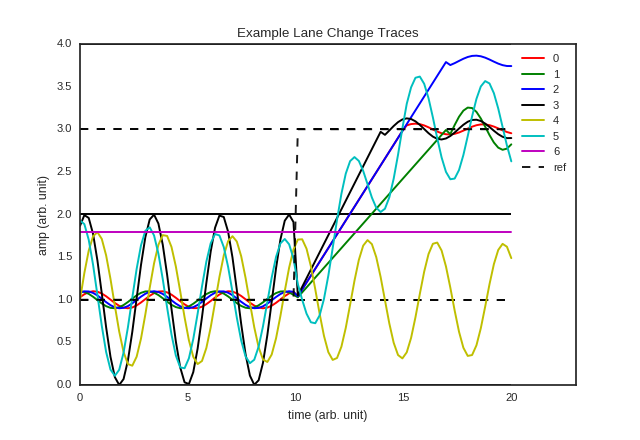$$\Rightarrow$$

# Slow Downs Revisited

$\square_{[\tau, 20]}(\text{car_speed} \leq h)$# Slow Downs Revisited

$\square_{[\tau, 20]}(\text{car_speed} \leq h)$# Outline

1. Motivating Example
2. Logical Lens $$\to$$ Logical Distance

Logical Lens $$\triangleq$$ Parametric Trace Property

3. Computing Logical Distance
4. Application to Highway 101 data.

# Real Highway Data Example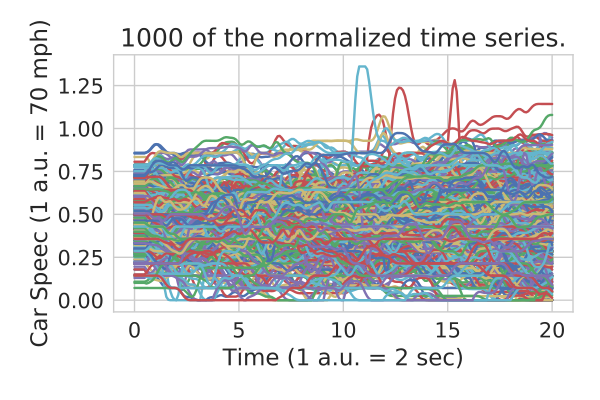• Want to detect traffic slow downs.
• Too much data to run logical distance on!!!!
• Many metric based learning algorithms are will require $O(\#\text{dataPoints}^2)$ queries.

Colyar, J., Halkias, J.: US highway 101 dataset. Federal Highway Administration (FHWA), Tech. Rep. FHWA-HRT-07-030 (2007)

# Outline

1. Motivating Example
2. Logical Lens $$\to$$ Coarse Logical Distance

3. Computing Logical Distance
4. Application to Highway 101 data.

# Coarse Logical Features# Coarse Logical FeaturesCompute intersections along a line.

# Coarse Logical FeaturesParameterize line between 0 and 1.

$$\gamma_i : \text{Data} \to [0, 1]$$.

# Coarse Logical FeaturesCan project to multiple lines.

# Outline

1. Motivating Example
2. Logical Lens $$\to$$ Logical Distance

Logical Lens $$\triangleq$$ Parametric Trace Property

3. Computing Logical Distance
4. Application to Highway 101 data.

# Real Highway Data Example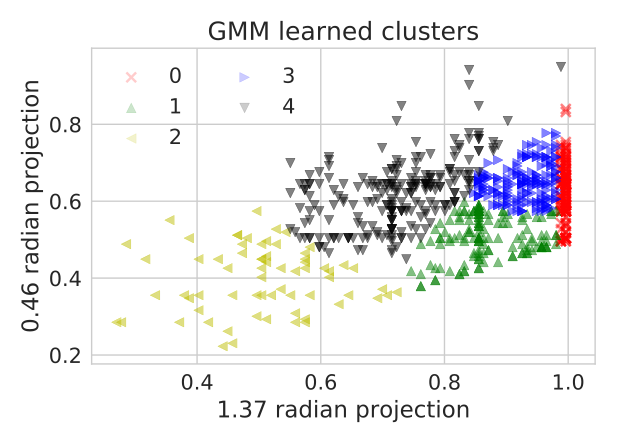1. Project onto two interior lines.
2. Label using Gaussian Mixture Model.
3. Label toy data to identify possible slow downs.

# Distance to Idealized Slowdown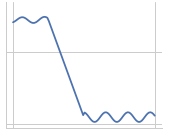Zoom in on cluster 4 by computing distances to idealized slow down.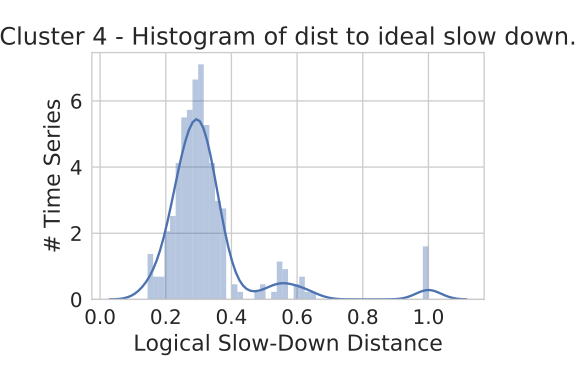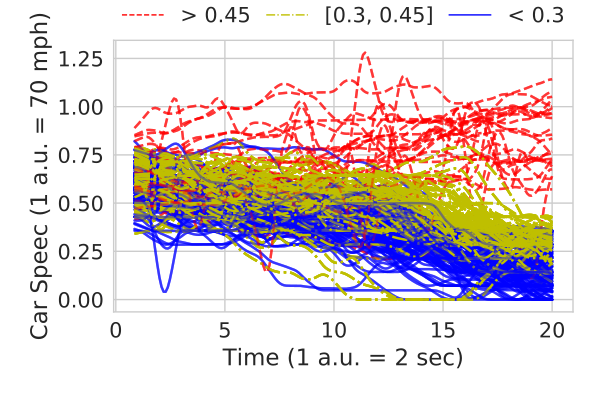# Extract Formula?

Labels $$\to$$ Parameter Ranges $$\to$$ Formula

Details in the CAV 2017 paper.

Logic-based Clustering and Learning for Time-Series Data, CAV 2017

# Slow Down FormulaExtracting a formula:

$\phi(\tau, h) \triangleq \square_{[\tau, 20]}(\text{car_speed} < h)$

$\psi_1 = \phi(0.25, 0.55) \wedge \neg \phi(0.38, 0.55) \wedge \neg \phi(0.25, 0.76)$

$\psi_2 = \phi(0.35, 0.17) \wedge \neg \phi(0.35, 0.31) \wedge \neg \phi(0.62, 0.17)$

$\text{slow_down} \triangleq \psi_1 \wedge \psi_2$

# Summary

1. Relax restrictions of our CAV 2017 work.
2. Learn "traffic slow-down" spec from simple logical lens.

# Future work

1. Performance improvements.
2. Kernel trick analog to learn better specifications.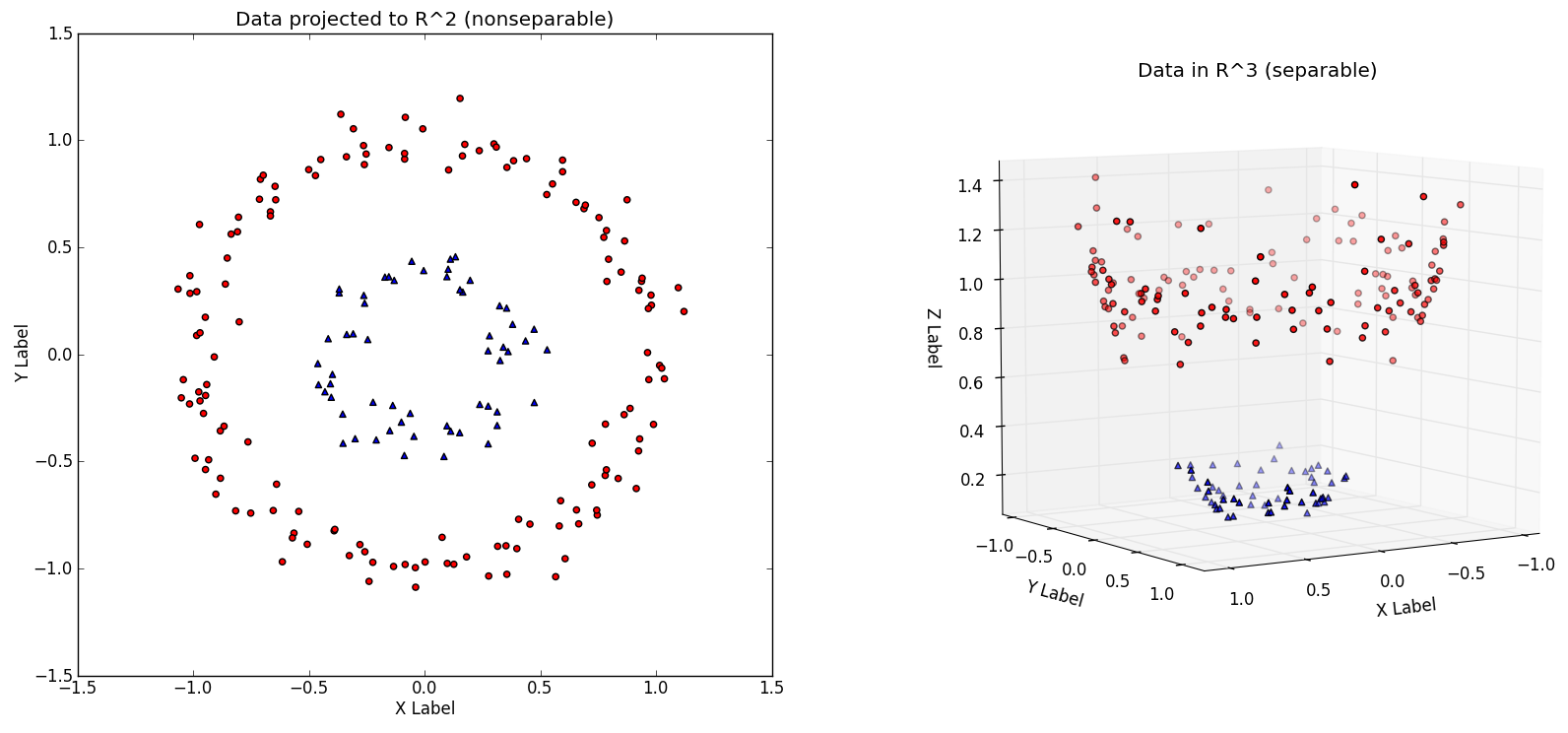# Questions?

Slides @ mjvc.me/RV2018

# A hammer looking for a nail?1. A lot of great tools to time-series learning: Dynamic Time Warping, Shaplets, PCA, ...
2. All of these techniques are fantastic!
3. But you know what's better? A good feature.

# Feature Engineering is King

"Coming up with features is difficult, time-consuming, requires expert knowledge. "Applied machine learning" is basically feature engineering."

- Andrew Ng,
"Machine Learning and AI via Brain simulations"

" …some machine learning projects succeed and some fail. What makes the difference? Easily the most important factor is the features used."

- Pedro Domingos,
"A Few Useful Things to Know about Machine Learning"

... or at least is was before Deep Learning

# Designing features is HARD

1. Need to embed non-trivial domain specific knowledge into the feature.
2. Domain specific knowledge is typically stuck in the head of a non-ML expert.
3. ML techniques can be sensitive to encoding of features.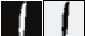This work focuses on try to make designing features slightly less hard (e.g., time series).

# Validity Domain

$\phi : \text{Data} \to \bigg ( {[0, 1]}^n \to \{0, 1\}\bigg)$

$V_\phi(x) \triangleq ~\text{preImg}_{\phi(x)}[\{1\}] = \{\theta \in {[0, 1]}^n~.~\phi(x)(\theta) = 1\}$# What's to come

(3/5) Derive a "logical distance" under this lens.$\phi(\tau, h) \triangleq \square_{[\tau, 20]}(\text{car_speed} \leq h)$

# What's to come

(4/5) Apply to real highway data.$\phi(\tau, h) \triangleq \square_{[\tau, 20]}(\text{car_speed} \leq h)$

# What's to come

(5/5) Characterize slow downs in this dataset.$\phi(\tau, h) \triangleq \square_{[\tau, 20]}(\text{car_speed} \leq h)$

$\begin{split}\psi_1 \triangleq \phi(0.25, 0.55) &\wedge \neg \phi(0.38, 0.55) \\&\wedge \neg \phi(0.25, 0.76)\end{split}$

$\begin{split}\psi_2 \triangleq \phi(0.35, 0.17) &\wedge \neg \phi(0.35, 0.31) \\&\wedge \neg \phi(0.62, 0.17)\end{split}$

$\text{slow_down} \triangleq \psi_1 \wedge \psi_2$

# Distance between Parameters

Infinity Norm: Let the distance between two parameters be the difference in their largest component, e.g,

$\text{height} \mapsto h, \text{weight} \mapsto w$ $d_\infty((h_1, w_1), (h_2, w_2)) \triangleq \max(|h_1 - h_2|, |w_1 - w_2|)$# Distance between Boundaries

Hausdorff Distance: Let $A$ and $B$ be two sets of parameters:

$d^H_\infty(A, B) \triangleq \max(\sup_{a \in A} \inf_{b \in B} d_\infty(a, b), \sup_{b \in B} \inf_{a \in A} d_\infty(a, b))$

# Distance between Boundaries

$d^H_\infty(A, B) \triangleq \max(\sup_{a \in A} \inf_{b \in B} d_\infty(a, b), \sup_{b \in B} \inf_{a \in A} d_\infty(a, b))$Interpretation: The curves "$$\epsilon$$-bisimulate" each other.

1. Two player game where player 1 selects a point, $a \in A$ and then player 2 selects a point $b\in B$.
2. Player 1 wants to maximize distance between and $a$ and $b$.
3. Player 2 wants to minimize the distance.
4. They then switch roles.